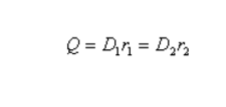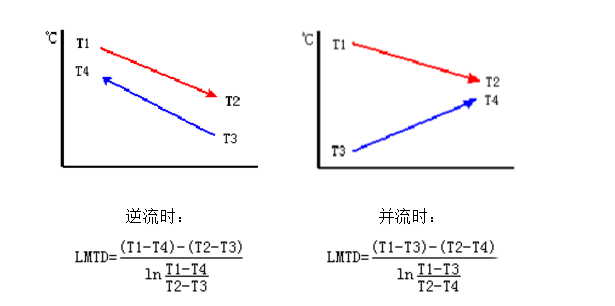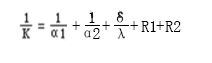(+86)-029-85200606       sales@guphe.comEnglish

# Calculation method of plate heat exchanger

Views:0     Author:Site Editor     Publish Time: 2020-05-05      Origin:Site

The calculation of plate heat exchanger is a relatively complicated process, and the currently popular methods are logarithmic average temperature difference method and NTU method. When computers were not popular, most manufacturers used approximate calculation parameters and flow rate-total heat transfer coefficient curve estimation methods. At present, more and more manufacturers use computer calculation, so that the process calculation of plate heat exchanger becomes fast, convenient and accurate. The following is a brief description of the general calculation method of the plate heat exchanger without phase transition. This method is a design calculation method based on the correlation between the heat transfer and pressure drop criteria.

## The following five parameters are necessary in the selection calculation of the plate heat exchanger:

Total heat transfer (unit: kW).

Inlet and outlet temperature of primary side and secondary side

Allowable pressure drop on the primary and secondary sides

Maximum working temperature

Maximum working pressure If the flow rate of the heat transfer medium is known, the specific heat capacity and the temperature difference between the inlet and outlet, the total heat transfer can be calculated.

Temperature

T1 = Hot side inlet temperature

T2 = Hot side outlet temperature

t1 = cold side inlet temperature

t2 = cold side outlet temperature

The heat flow balance formula reflects the mutual relationship between the temperature changes of the two fluids during heat exchange. Under the condition of good heat insulation of the heat exchanger and no heat loss, the heat flow balance relationship for the steady state heat transfer process is:

(Heat flow rate released by hot fluid) = (heat flow rate absorbed by cold fluid)

In the heat balance calculation, the expression of the heat transfer process with and without phase change is different.

(1) Heat transfer process without phase change

Where

Q ---- The heat flux absorbed by cold fluid or released by hot fluid, W;

mh, mc ----- mass flow of hot and cold fluid, kg / s;

Cph, Cpc ------ The specific pressure heat capacity of hot and cold fluid, kJ / (kg · K);

T1, t1 ------ Hot and cold fluid inlet temperature, K;

T2, t2 ------ outlet temperature of hot and cold fluid, K.

(2) Heat transfer process with phase change

During the heat exchange between the two streams, one of the streams undergoes a phase change, such as steam condensation or liquid boiling, and the heat flow balance formula is:

Has a phase change on one sideBoth sides of the stream have phase changes, such as condensing heat transfer process on the other sideWhere

r, r1, r2 -------- logistics phase change heat, J / kg;

D, D1, D2 -------- Flow rate of phase change substance, kg / s.

For the calculation of heat flow when the supercooled or superheated stream undergoes a phase change, the summation should be calculated in accordance with the above method.

Log Mean Temperature Difference (LMTD)

The logarithmic average temperature difference is the power of heat transfer of the heat exchanger. The size of the logarithmic average temperature difference directly relates to the degree of heat transfer of the heat exchanger. In some special cases, the logarithmic average temperature difference cannot be calculated. In this case, the arithmetic average temperature difference is used instead of the logarithmic average temperature difference. The calculation method of the medium logarithmic average temperature difference between the countercurrent and the cocurrent conditions is different. In some special cases, the arithmetic average temperature difference is used instead of the logarithmic average temperature difference.

Analysis and calculation method of heat transfer coefficient and resistance of plate heat exchangerHot long (F)

The heat length is related to the temperature difference on one side and the logarithmic average temperature difference. F = dt / LMTD

The heat transfer density, viscosity, specific heat capacity and thermal conductivity affected by the physical properties of the following four media

Total heat transfer coefficient

The total heat transfer coefficient is a parameter used to measure the heat transfer resistance of the heat exchanger. The heat transfer resistance is mainly composed of factors such as the material and thickness of the heat transfer plate, dirt and the fluid itself. Unit: W / m2 ℃ or kcal / h, m2 ℃.

Pressure drop

The pressure drop directly affects the size of the plate heat exchanger. If there is a larger allowable pressure drop, it may reduce the cost of the heat exchanger, but it will lose the power of the pump and increase the operating cost. Under normal circumstances, in the case of water-to-water heat exchange, the allowable pressure drop is generally acceptable at 20-100KPa.

Fouling coefficient

Compared with shell and tube heat exchangers, the flow of water in plate heat exchangers is in a state of high turbulence, and the fouling coefficient of the same medium is much smaller than that of plate heat exchangers. In the case where the scale factor of water cannot be determined, 10% of the surplus can be retained in the calculation.

Calculation method The thermal load can be expressed by the following formula:

Q = m · cp · dt

Q = k · A · LMTD

m = mass flow rate (kg / s)

cp = specific heat (kJ / kg ℃)

dt = temperature difference between inlet and outlet of medium (℃)

K = total heat transfer coefficient (W / m2 ℃)

A = heat transfer area (m2)

LMTD = logarithmic mean temperature difference

The total heat transfer coefficient is calculated by the following formula:

Analysis and calculation method of heat transfer coefficient and resistance of plate heat exchangeramong them:

K = Total heat transfer coefficient (W / m2 ℃)

α1 = One-time measured heat transfer coefficient (W / m2 ℃)

α2 = One-time measured heat transfer coefficient (W / m2 ℃)

δ = Thickness of heat transfer plate (m)

λ = The thermal conductivity of the plate (W / m ℃)

R1 and R2 are the fouling coefficients on both sides (m2 ℃ / W)

α1 and α2 can be obtained by Nusselt criterion.

### Heat transfer efficiency and heat transfer unit number method

In the heat transfer calculation, the heat transfer rate equation and heat flow balance formula relate the parameters of the heat exchanger and the heat exchange stream. When the flow rate, inlet and outlet temperature of the process stream are known, the average heat transfer temperature difference △ tm and heat flow Q can be calculated according to the method described above, so as to obtain the required heat transfer area A. Such problems are mentioned above. And the design calculation problem.

However, when the flow rate, inlet temperature, heat transfer area, and heat transfer coefficient K of the two streams are given, it is difficult to directly determine the outlet temperature of the two fluids using analytical methods. Often need to use trial and error method to solve. This type of problem is the operational computing problem mentioned earlier. In this regard, if the heat transfer efficiency and heat transfer unit number method derived from Case and London in 1955 are adopted, the solution can be obtained conveniently without trial and error.

Form Name